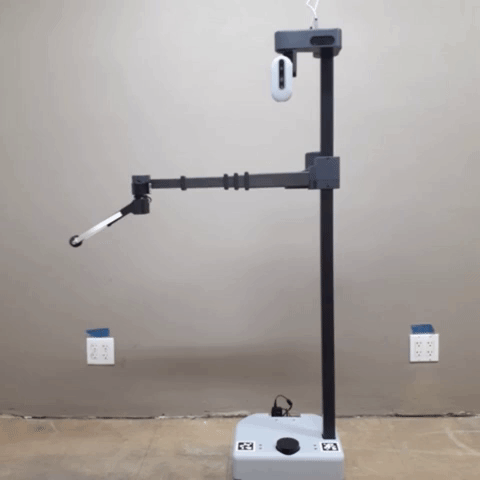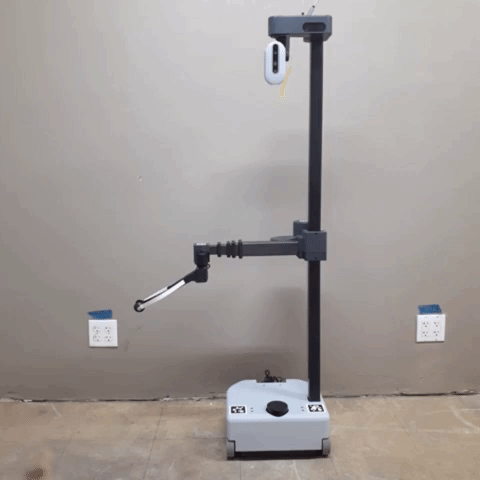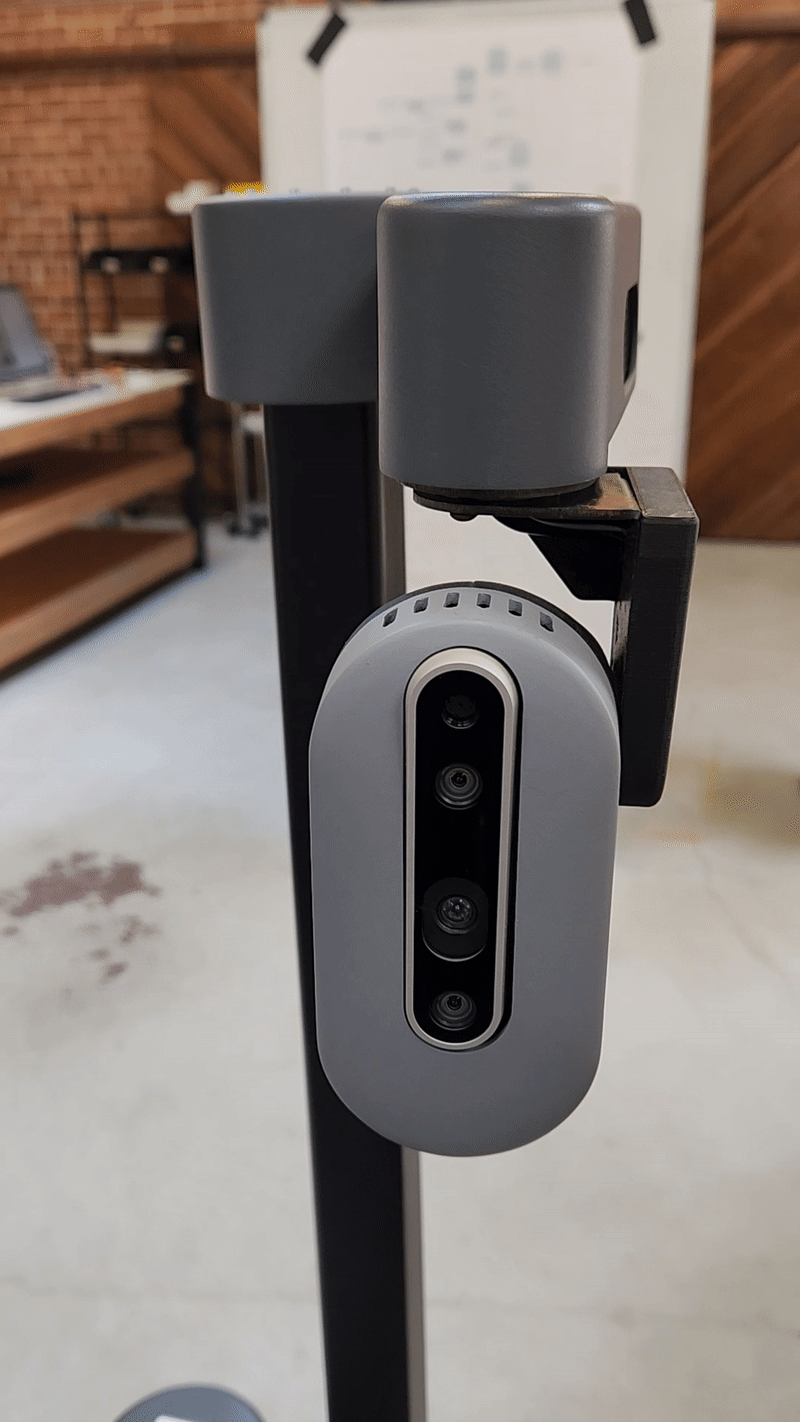## FollowJointTrajectory Commands

Stretch ROS driver offers a `FollowJointTrajectory` action service for its arm. Within this tutorial, we will have a simple FollowJointTrajectory command sent to a Stretch robot to execute.

## Stow Command ExampleBegin by running the following command in a terminal.

``````roslaunch stretch_core stretch_driver.launch
``````

In a new terminal, switch the mode to `position` mode using a rosservice call. Then run the stow command node.

``````rosservice call /switch_to_position_mode
cd catkin_ws/src/stretch_tutorials/src/
python3 stow_command.py
``````

This will send a `FollowJointTrajectory` command to stow Stretch's arm.

### The Code

``````#!/usr/bin/env python3

import rospy
from control_msgs.msg import FollowJointTrajectoryGoal
from trajectory_msgs.msg import JointTrajectoryPoint
import hello_helpers.hello_misc as hm
import time

class StowCommand(hm.HelloNode):
'''
A class that sends a joint trajectory goal to stow the Stretch's arm.
'''
def __init__(self):
hm.HelloNode.__init__(self)

def issue_stow_command(self):
'''
Function that makes an action call and sends stow position goal.
:param self: The self reference.
'''
stow_point = JointTrajectoryPoint()
stow_point.time_from_start = rospy.Duration(0.000)
stow_point.positions = [0.2, 0.0, 3.4]

trajectory_goal = FollowJointTrajectoryGoal()
trajectory_goal.trajectory.joint_names = ['joint_lift', 'wrist_extension', 'joint_wrist_yaw']
trajectory_goal.trajectory.points = [stow_point]

self.trajectory_client.send_goal(trajectory_goal)
self.trajectory_client.wait_for_result()

def main(self):
'''
Function that initiates stow_command function.
:param self: The self reference.
'''
hm.HelloNode.main(self, 'stow_command', 'stow_command', wait_for_first_pointcloud=False)
self.issue_stow_command()
time.sleep(2)

if __name__ == '__main__':
try:
node = StowCommand()
node.main()
except KeyboardInterrupt:
``````

### The Code Explained

Now let's break the code down.

``````#!/usr/bin/env python3
``````

Every Python ROS Node will have this declaration at the top. The first line makes sure your script is executed as a Python3 script.

``````import rospy
from control_msgs.msg import FollowJointTrajectoryGoal
from trajectory_msgs.msg import JointTrajectoryPoint
import hello_helpers.hello_misc as hm
import time
``````

You need to import `rospy` if you are writing a ROS Node. Import the `FollowJointTrajectoryGoal` from the control_msgs.msg package to control the Stretch robot. Import `JointTrajectoryPoint` from the trajectory_msgs package to define robot trajectories. The hello_helpers package consists of a module that provides various Python scripts used across stretch_ros. In this instance, we are importing the `hello_misc` script.

``````class StowCommand(hm.HelloNode):
def __init__(self):
hm.HelloNode.__init__(self)
``````

The `StowCommand` class inherits the `HelloNode` class from `hm` and is initialized.

``````def issue_stow_command(self):
stow_point = JointTrajectoryPoint()
stow_point.time_from_start = rospy.Duration(0.000)
stow_point.positions = [0.2, 0.0, 3.4]
``````

The `issue_stow_command()` is the name of the function that will stow Stretch's arm. Within the function, we set stow_point as a `JointTrajectoryPoint`and provide desired positions (in meters). These are the positions of the lift, wrist extension, and yaw of the wrist, respectively. These are defined next.

``````    trajectory_goal = FollowJointTrajectoryGoal()
trajectory_goal.trajectory.joint_names = ['joint_lift', 'wrist_extension', 'joint_wrist_yaw']
trajectory_goal.trajectory.points = [stow_point]
``````

Set `trajectory_goal` as a `FollowJointTrajectoryGoal` and define the joint names as a list. Then `trajectory_goal.trajectory.points` is defined by the positions set in `stow_point`. Specify the coordinate frame that we want (base_link) and set the time to be now.

``````self.trajectory_client.send_goal(trajectory_goal)
self.trajectory_client.wait_for_result()
``````

Make the action call and send the goal. The last line of code waits for the result before it exits the python script.

``````def main(self):
hm.HelloNode.main(self, 'stow_command', 'stow_command', wait_for_first_pointcloud=False)
self.issue_stow_command()
time.sleep(2)
``````

Create a function, `main()`, to set up the `hm.HelloNode` class and issue the stow command.

``````if __name__ == '__main__':
try:
node = StowCommand()
node.main()
except KeyboardInterrupt:
``````

Declare object, node, from the `StowCommand()` class. Then run the `main()` function.

## Multipoint Command ExampleBegin by running the following command in a terminal:

``````roslaunch stretch_core stretch_driver.launch
``````

In a new terminal, switch the mode to `position` mode using a rosservice call. Then run the multipoint command node.

``````rosservice call /switch_to_position_mode
cd catkin_ws/src/stretch_tutorials/src/
python3 multipoint_command.py
``````

This will send a list of `JointTrajectoryPoint` message types to move Stretch's arm.

### The Code

``````#!/usr/bin/env python3

import rospy
import time
from control_msgs.msg import FollowJointTrajectoryGoal
from trajectory_msgs.msg import JointTrajectoryPoint
import hello_helpers.hello_misc as hm

class MultiPointCommand(hm.HelloNode):
"""
A class that sends multiple joint trajectory goals to the stretch robot.
"""
def __init__(self):
hm.HelloNode.__init__(self)

def issue_multipoint_command(self):
"""
Function that makes an action call and sends multiple joint trajectory goals
to the joint_lift, wrist_extension, and joint_wrist_yaw.
:param self: The self reference.
"""
point0 = JointTrajectoryPoint()
point0.positions = [0.2, 0.0, 3.4]
point0.velocities = [0.2, 0.2, 2.5]
point0.accelerations = [1.0, 1.0, 3.5]

point1 = JointTrajectoryPoint()
point1.positions = [0.3, 0.1, 2.0]

point2 = JointTrajectoryPoint()
point2.positions = [0.5, 0.2, -1.0]

point3 = JointTrajectoryPoint()
point3.positions = [0.6, 0.3, 0.0]

point4 = JointTrajectoryPoint()
point4.positions = [0.8, 0.2, 1.0]

point5 = JointTrajectoryPoint()
point5.positions = [0.5, 0.1, 0.0]

trajectory_goal = FollowJointTrajectoryGoal()
trajectory_goal.trajectory.joint_names = ['joint_lift', 'wrist_extension', 'joint_wrist_yaw']
trajectory_goal.trajectory.points = [point0, point1, point2, point3, point4, point5]

self.trajectory_client.send_goal(trajectory_goal)
rospy.loginfo('Sent list of goals = {0}'.format(trajectory_goal))
self.trajectory_client.wait_for_result()

def main(self):
"""
Function that initiates the multipoint_command function.
:param self: The self reference.
"""
hm.HelloNode.main(self, 'multipoint_command', 'multipoint_command', wait_for_first_pointcloud=False)
self.issue_multipoint_command()
time.sleep(2)

if __name__ == '__main__':
try:
node = MultiPointCommand()
node.main()
except KeyboardInterrupt:
``````

### The Code Explained

Seeing that there are similarities between the multipoint and stow command nodes, we will only break down the different components of the `multipoint_command` node.

``````point0 = JointTrajectoryPoint()
point0.positions = [0.2, 0.0, 3.4]
``````

Set `point0` as a `JointTrajectoryPoint`and provide desired positions. These are the positions of the lift, wrist extension, and yaw of the wrist, respectively. The lift and wrist extension positions are expressed in meters, whereas the wrist yaw is in radians.

``````point0.velocities = [0.2, 0.2, 2.5]
``````
Provide the desired velocity of the lift (m/s), wrist extension (m/s), and wrist yaw (rad/s) for `point0`.

``````point0.accelerations = [1.0, 1.0, 3.5]
``````

Provide desired accelerations of the lift (m/s^2), wrist extension (m/s^2), and wrist yaw (rad/s^2).

Note

The lift and wrist extension can only go up to 0.2 m/s. If you do not provide any velocities or accelerations for the lift or wrist extension, then they go to their default values. However, the Velocity and Acceleration of the wrist yaw will stay the same from the previous value unless updated.

``````trajectory_goal = FollowJointTrajectoryGoal()
trajectory_goal.trajectory.joint_names = ['joint_lift', 'wrist_extension', 'joint_wrist_yaw']
trajectory_goal.trajectory.points = [point0, point1, point2, point3, point4, point5]
``````

Set `trajectory_goal` as a `FollowJointTrajectoryGoal` and define the joint names as a list. Then `trajectory_goal.trajectory.points` is defined by a list of the 6 points. Specify the coordinate frame that we want (base_link) and set the time to be now.

## Single Joint ActuatorYou can also actuate a single joint for the Stretch. Below is the list of joints and their position limit.

``````############################# JOINT LIMITS #############################
joint_lift:      lower_limit =  0.15,  upper_limit =  1.10  # in meters
wrist_extension: lower_limit =  0.00,  upper_limit =  0.50  # in meters
joint_wrist_yaw: lower_limit = -1.75,  upper_limit =  4.00  # in radians
joint_gripper_finger_left:  lower_limit = -0.35,  upper_limit =  0.165  # in radians

# INCLUDED JOINTS IN POSITION MODE
translate_mobile_base: No lower or upper limit. Defined by a step size in meters
rotate_mobile_base:    No lower or upper limit. Defined by a step size in radians
########################################################################
``````

Begin by running the following command in a terminal.

``````roslaunch stretch_core stretch_driver.launch
``````

In a new terminal, switch the mode to `position` mode using a rosservice call. Then run the single joint actuator node.

``````rosservice call /switch_to_position_mode
cd catkin_ws/src/stretch_tutorials/src/
python3 single_joint_actuator.py
``````
This will send a list of `JointTrajectoryPoint` message types to move Stretch's arm.

The joint, `joint_gripper_finger_left`, is only needed when actuating the gripper.

### The Code

``````#!/usr/bin/env python3

import rospy
import time
from control_msgs.msg import FollowJointTrajectoryGoal
from trajectory_msgs.msg import JointTrajectoryPoint
import hello_helpers.hello_misc as hm

class SingleJointActuator(hm.HelloNode):
"""
A class that sends multiple joint trajectory goals to a single joint.
"""
def __init__(self):
hm.HelloNode.__init__(self)

def issue_command(self):
"""
Function that makes an action call and sends joint trajectory goals
to a single joint
:param self: The self reference.
"""
trajectory_goal = FollowJointTrajectoryGoal()

point0 = JointTrajectoryPoint()
point0.positions = [0.65]

# point1 = JointTrajectoryPoint()
# point1.positions = [0.5]

trajectory_goal.trajectory.points = [point0]#, point1]
self.trajectory_client.send_goal(trajectory_goal)
self.trajectory_client.wait_for_result()

def main(self):
"""
Function that initiates the issue_command function.
:param self: The self reference.
"""
hm.HelloNode.main(self, 'issue_command', 'issue_command', wait_for_first_pointcloud=False)
self.issue_command()
time.sleep(2)

if __name__ == '__main__':
try:
node = SingleJointActuator()
node.main()
except KeyboardInterrupt:
``````

### The Code Explained

Since the code is quite similar to the `multipoint_command` code, we will only review the parts that differ.

Now let's break the code down.

``````trajectory_goal = FollowJointTrajectoryGoal()
``````

Here we only input the joint name that we want to actuate. In this instance, we will actuate the `joint_head_pan`.

``````point0 = JointTrajectoryPoint()
point0.positions = [0.65]

# point1 = JointTrajectoryPoint()
# point1.positions = [0.5]
``````

Set `point0` as a `JointTrajectoryPoint`and provide the desired position. You also have the option to send multiple point positions rather than one.

``````trajectory_goal.trajectory.points = [point0]#, point1]
Set `trajectory_goal` as a `FollowJointTrajectoryGoal` and define the joint names as a list. Then `trajectory_goal.trajectory.points` set by your list of points. Specify the coordinate frame that we want (base_link) and set the time to be now.